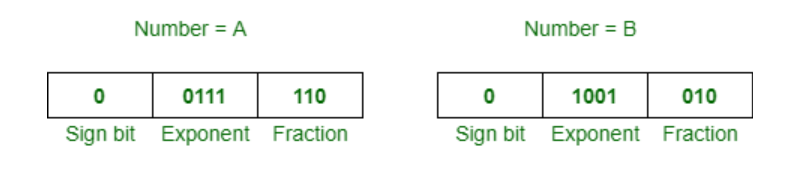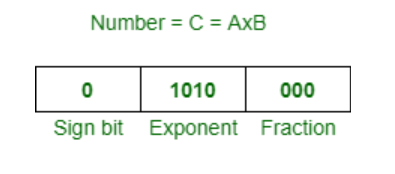# Multiplying Floating Point Numbers

Prerequisite – IEEE Standard 754 Floating Point Numbers
Problem:-
Here, we have discussed an algorithm to multiply two floating point numbers, x and y.

Algorithm:-

1. Convert these numbers in scientific notation, so that we can explicitly represent hidden 1.
2. Let ‘a’ be the exponent of x and ‘b’ be the exponent of y.
3. Assume resulting exponent c = a+b. It can be adjusted after the next step.
4. Multiply mantissa of x to mantissa of y. Call this result m.
5. If m does not have a single 1 left of radix point, then adjust radix point so it does, and adjust exponent c to compensate.
6. Add sign bits, mod 2, to get sign of resulting multiplication.
7. Convert back to one byte floating point representation, truncating bits if needed.

Note :
Negative values are simple to take care of in floating point multiplication. Treat sign bit as 1 bit unsigned binary, add mod 2. This is the same as XORing the sign bit.

Example :-
Suppose you want to multiply following two numbers:Now, these are steps according to above algorithm:

1. Given, A = 1.11 x 2^0 and B = 1.01 x 2^2
2. So, exponent c = a + b = 0 + 2 = 2 is the resulting exponent.
3. Now, multiply 1.11 by 1.01, so result will be 10.0011
4. We need to normalize 10.0011 to 1.00011 and adjust exponent 1 by 3 appropriately.
5. Resulting sign bit 0 (XOR) 0 = 0, means positive.
6. Now, truncate and normalite it 1.00011 x 2^3 to 1.000 x 2^3.

Therefore, resultant number is,Similarly, we can multiply other floating point numbers.

Don’t stop now and take your learning to the next level. Learn all the important concepts of Data Structures and Algorithms with the help of the most trusted course: DSA Self Paced. Become industry ready at a student-friendly price.

My Personal Notes arrow_drop_upIf you like GeeksforGeeks and would like to contribute, you can also write an article using contribute.geeksforgeeks.org or mail your article to contribute@geeksforgeeks.org. See your article appearing on the GeeksforGeeks main page and help other Geeks.

Please Improve this article if you find anything incorrect by clicking on the "Improve Article" button below.